## 1. 欧氏距离(Euclidean Distance)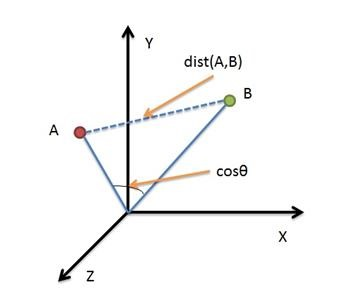• 二维平面上点a(x1,y1)与b(x2,y2)间的欧氏距离: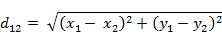• 三维空间点a(x1,y1,z1)与b(x2,y2,z2)间的欧氏距离: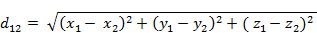• n维空间点a(x11,x12,…,x1n)与b(x21,x22,…,x2n)间的欧氏距离（两个n维向量）：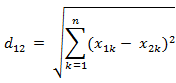• Matlab计算欧氏距离:

Matlab计算距离使用pdist函数。若X是一个m×n的矩阵，则pdist(X)将X矩阵每一行作为一个n维行向量，然后计算这m个向量两两间的距离。

`````` X=[1 1;2 2;3 3;4 4];
d=pdist(X,'euclidean')
d=
1.4142    2.8284    4.2426    1.4142    2.8284    1.4142 ``````

## 2. 曼哈顿距离(Manhattan Distance)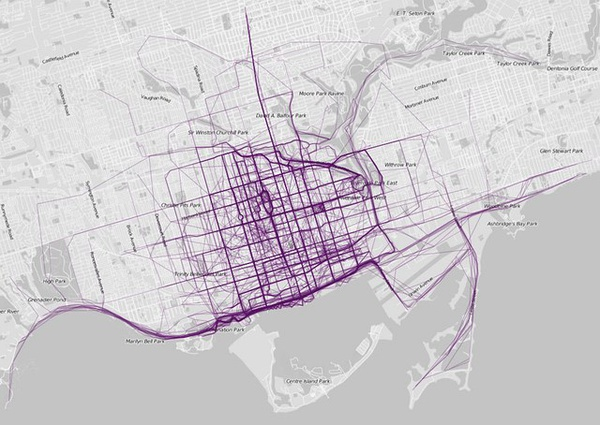• 二维平面两点a(x1,y1)与b(x2,y2)间的曼哈顿距离：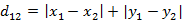• n维空间点a(x11,x12,…,x1n)与b(x21,x22,…,x2n)的曼哈顿距离：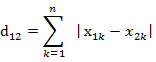• Matlab计算曼哈顿距离：

`````` X=[1 1;2 2;3 3;4 4];
d=pdist(X,'cityblock')
d=
2     4     6     2     4     2 ``````

## 3. 切比雪夫距离 (Chebyshev Distance)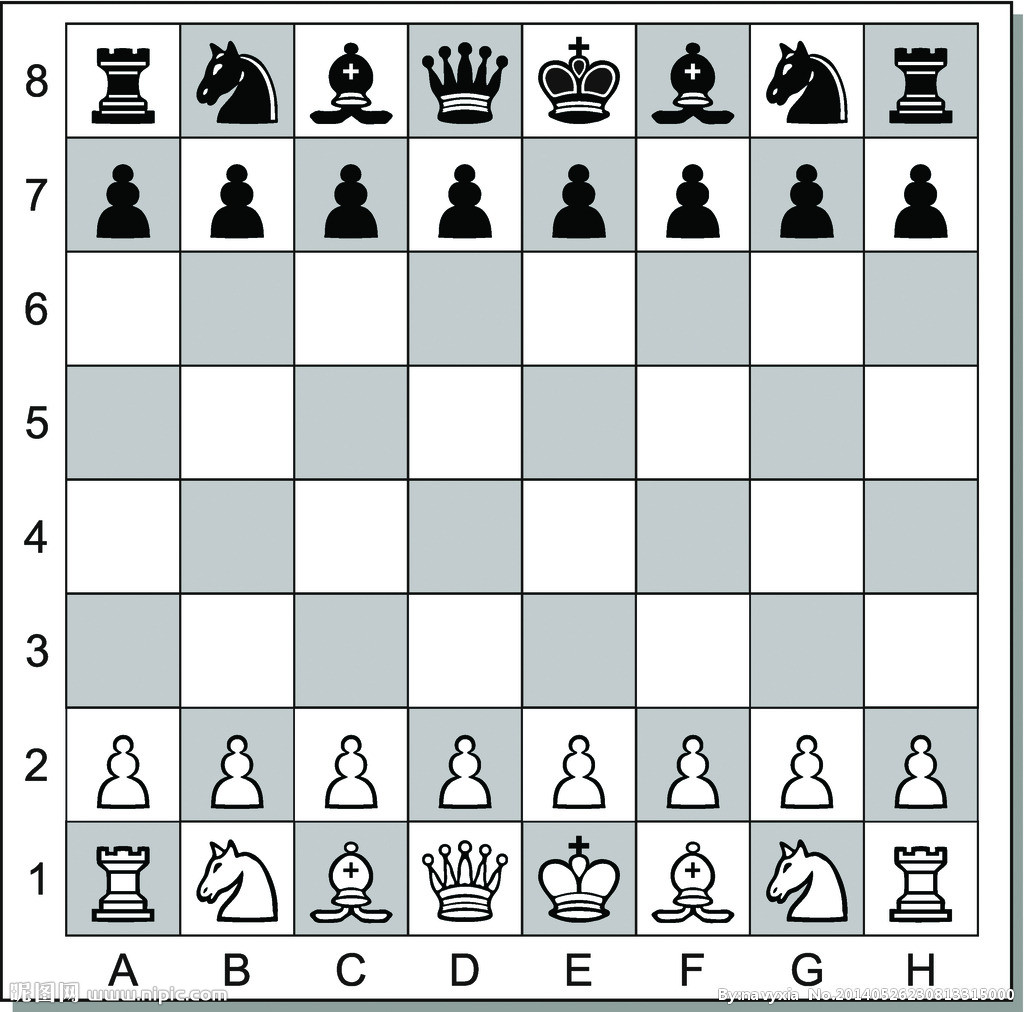• 二维平面两点a(x1,y1)与b(x2,y2)间的切比雪夫距离：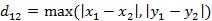• n维空间点a(x11,x12,…,x1n)与b(x21,x22,…,x2n)的切比雪夫距离：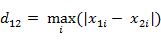• Matlab计算切比雪夫距离：

`````` X=[1 1;2 2;3 3;4 4];
d=pdist(X,'chebychev')
d=
1     2     3     1     2     1 ``````

## 4. 闵可夫斯基距离(Minkowski Distance)

• 闵氏距离定义：
• 两个n维变量a(x11,x12,…,x1n)与b(x21,x22,…,x2n)间的闵可夫斯基距离定义为：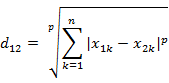• 闵氏距离，包括曼哈顿距离、欧氏距离和切比雪夫距离都存在明显的缺点。
• e.g. 二维样本(身高[单位:cm],体重[单位:kg]),现有三个样本：a(180,50)，b(190,50)，c(180,60)。那么a与b的闵氏距离（无论是曼哈顿距离、欧氏距离或切比雪夫距离）等于a与c的闵氏距离。但实际上身高的10cm并不能和体重的10kg划等号。
• 闵氏距离的缺点：
• (1)将各个分量的量纲(scale)，也就是“单位”相同的看待了;
• (2)未考虑各个分量的分布（期望，方差等）可能是不同的。

• Matlab计算闵氏距离（以p=2的欧氏距离为例）：

`````` X=[1 1;2 2;3 3;4 4];
d=pdist(X,'minkowski',2)
d=
1.4142    2.8284    4.2426    1.4142    2.8284    1.4142 ``````

## 5. 标准化欧氏距离 (Standardized Euclidean Distance)

定义： 标准化欧氏距离是针对欧氏距离的缺点而作的一种改进。标准欧氏距离的思路：既然数据各维分量的分布不一样，那先将各个分量都“标准化”到均值、方差相等。假设样本集X的均值(mean)为m，标准差(standard deviation)为s，X的“标准化变量”表示为：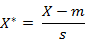• 标准化欧氏距离公式：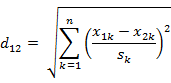• Matlab计算标准化欧氏距离（假设两个分量的标准差分别为0.5和1）：

`````` X=[1 1;2 2;3 3;4 4];
d=pdist(X,'seuclidean',[0.5,1])
d=
2.2361    4.4721    6.7082    2.2361    4.4721    2.2361 ``````

## 6. 马氏距离(Mahalanobis Distance)

马氏距离的引出：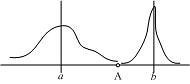• 概念：马氏距离是基于样本分布的一种距离。物理意义就是在规范化的主成分空间中的欧氏距离。所谓规范化的主成分空间就是利用主成分分析对一些数据进行主成分分解。再对所有主成分分解轴做归一化，形成新的坐标轴。由这些坐标轴张成的空间就是规范化的主成分空间。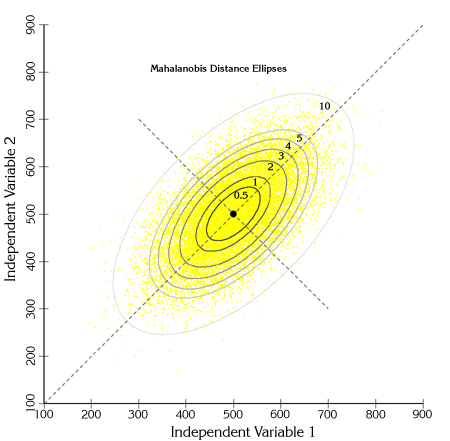• 定义：有M个样本向量X1~Xm，协方差矩阵记为S，均值记为向量μ，则其中样本向量X到μ的马氏距离表示为：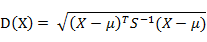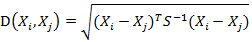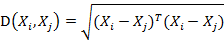• 欧式距离&马氏距离：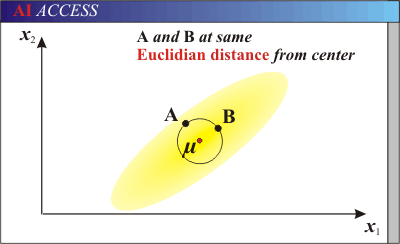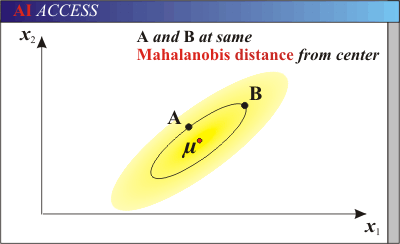• 马氏距离的特点：
• 量纲无关，排除变量之间的相关性的干扰；
• 马氏距离的计算是建立在总体样本的基础上的，如果拿同样的两个样本，放入两个不同的总体中，最后计算得出的两个样本间的马氏距离通常是不相同的，除非这两个总体的协方差矩阵碰巧相同；
• 计算马氏距离过程中，要求总体样本数大于样本的维数，否则得到的总体样本协方差矩阵逆矩阵不存在，这种情况下，用欧式距离计算即可。
• Matlab计算马氏距离：

`````` X=[1 2;1 3;2 2;3 1];
d=pdist(X,'mahal')
d=
2.3452    2.0000    2.3452    1.2247    2.4495    1.2247 ``````

## 7. 余弦距离(Cosine Distance)

• 二维空间中向量A(x1,y1)与向量B(x2,y2)的夹角余弦公式：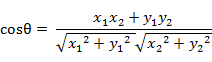• 两个n维样本点a(x11,x12,…,x1n)和b(x21,x22,…,x2n)的夹角余弦为：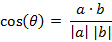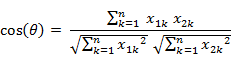• Matlab计算夹角余弦（Matlab中的pdist(X, ‘cosine’)得到的是1减夹角余弦的值）：

`````` X=[1 1;1 2;2 5;1 -4];
d=1-pdist(X,'cosine')
d=
0.9487    0.9191   -0.5145    0.9965   -0.7593   -0.8107 ``````

## 8. 汉明距离(Hamming Distance)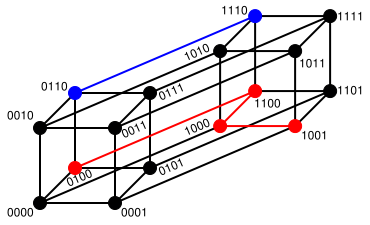• 定义：两个等长字符串s1与s2的汉明距离为：将其中一个变为另外一个所需要作的最小字符替换次数。例如:

`` The Hamming distance between "1011101" and "1001001" is 2. The Hamming distance between "2143896" and "2233796" is 3. The Hamming distance between "toned" and "roses" is 3. ``
• 汉明重量：是字符串相对于同样长度的零字符串的汉明距离，也就是说，它是字符串中非零的元素个数：对于二进制字符串来说，就是 1 的个数，所以 11101 的汉明重量是 4。因此，如果向量空间中的元素a和b之间的汉明距离等于它们汉明重量的差a-b。

• 应用：汉明重量分析在包括信息论、编码理论、密码学等领域都有应用。比如在信息编码过程中，为了增强容错性，应使得编码间的最小汉明距离尽可能大。但是，如果要比较两个不同长度的字符串，不仅要进行替换，而且要进行插入与删除的运算，在这种场合下，通常使用更加复杂的编辑距离等算法。

• Matlab计算汉明距离（Matlab中2个向量之间的汉明距离的定义为2个向量不同的分量所占的百分比）：

`````` X=[0 1 1;1 1 2;1 5 2];
d=pdist(X,'hamming')
d=
0.6667    1.0000    0.3333 ``````

## 9. 杰卡德距离(Jaccard Distance)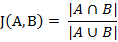• 杰卡德距离(Jaccard Distance)：与杰卡德相似系数相反，用两个集合中不同元素占所有元素的比例来衡量两个集合的区分度：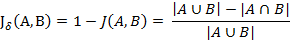• Matlab计算杰卡德距离（Matlab中将杰卡德距离定义为不同的维度的个数占“非全零维度”的比例）：

`````` X=[1 1 0;1 -1 0;-1 1 0];
d=pdist(X,'jaccard')
d=
0.5000    0.5000    1.0000 ``````

## 10. 相关距离(Correlation distance)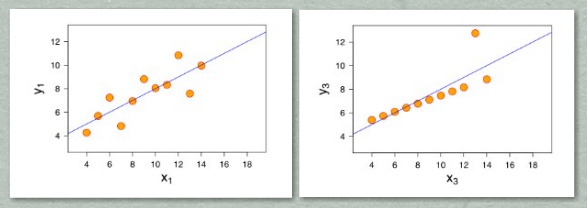• 相关系数：是衡量随机变量X与Y相关程度的一种方法，相关系数的取值范围是[-1,1]。相关系数的绝对值越大，则表明X与Y相关度越高。当X与Y线性相关时，相关系数取值为1（正线性相关）或-1（负线性相关）：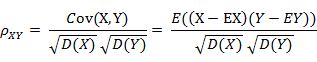• 相关距离：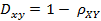• Matlab计算相关系数与相关距离：

`````` X=[1 2 3 4;3 8 7 6];
c=corrcoef(X') %返回相关系数矩阵
d=pdist(X,'correlation') %返回相关距离
c=
1.0000    0.4781
0.4781    1.0000
d=
0.5219 ``````

## 11. 信息熵(Information Entropy)

以上的距离度量方法度量的皆为两个样本（向量）之间的距离，而信息熵描述的是整个系统内部样本之间的一个距离，或者称之为系统内样本分布的集中程度（一致程度）、分散程度、混乱程度（不一致程度）。系统内样本分布越分散(或者说分布越平均)，信息熵就越大。分布越有序（或者说分布越集中），信息熵就越小。• 信息熵的由来：请参考博客：XXXXXXXX。

• 计算给定的样本集X的信息熵的公式：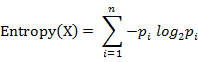n：样本集X的分类数

pi：X中第 i 类元素出现的概率